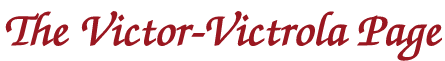An Introduction to Vibro-Acoustics

This page provides a general discussion to the topic of Vibro-Acoustics, for those who have had little or no previous exposure to this field. I will attempt to avoid extensive mathematics or derivations to the extent possible, and hopefully will provide some basic background to this topic in terms that are easily understood. Most people are familiar with the terms "sound" and "vibration". These two phenomena are inseparably linked. Sound is always caused by vibration. We can feel the vibration when we touch the surface of a loud stereo speaker or almost any rotating machine. Some structure or object must be vibrating in order for sound to occur.

Another "ingredient" for sound is the presence of some elastic medium for the sound to travel through. It can be water, solid structures, or most commonly, air. When an object vibrates in air, it disturbs local air molecules. These molecules bump into one another and cause a tiny change in the instantaneous air pressure that we hear as sound.

Vibration is measured as the motion of a structure as a function of time. Sound on the other hand, is measured as the minute change in pressure that is caused by the motion of the air molecules. When an object vibrates, only a tiny fraction of the vibrational energy is converted into sound; some surfaces are more efficient sound radiators than others, resulting in more sound energy being generated.

In recent years, measurement instrumentation for sound and vibration has improved dramatically. Precision microphones are used to measure sound characteristics, and devices called accelerometers are used to measure structural vibration. In addition, laser beams can now be used to monitor the motion of a small surface without the need to physically connect a sensor to the surface itself. These measurement systems, along with the extremely sophisticated signal processing capabilities of computerized analyzers allow us to take a very detailed look at the performance of vibro-acoustic devices.

The Propagation of Sound

On a large scale, sound radiates from the vibrating source in all directions. The effect is similar to dropping a stone into a quiet pond; the sound pressure waves move out in concentric rings from the center source. However, this analogy is not totally accurate. In order to understand how sound actually "moves", it is necessary to look at the air molecules themselves. If we look at a single air molecule that is being excited by sound (Figure 1) we can see that the molecule only oscillates about its equilibrium position. It simply moves back and forth; it does not travel along a path from point to point in the listening room. However, the molecule does strike neighboring molecules, and propagates the disturbance. The neighboring molecules now oscillate about their center points, having been excited by the original molecule. This exchange of energy from molecule to molecule is what we hear as sound. It is actually tiny fluctuations in pressure caused by this molecular motion.Figure 1. Air molecule motion. Each molecule moves back and forth, bumping into adjoining molecules, and then returning to its center point.

When a solid object begins vibrating, it moves back and forth a small amount about a center point. The motion compresses local air molecules as the object moves one way, and decompresses them when it moves back the other way (see Figure 2). The compressed molecules bump into the adjoining molecules, and these neighbors become compressed, likewise squeezing against their neighbors. With each impact, a small amount of energy is lost, so this process continues in chain-reaction style, slowly losing energy until it fades away. Thus, the farther you are away from a sound source, the less volume you hear. Looking at this process on a broader scale, the pressure moves out from the source in the form of a wave.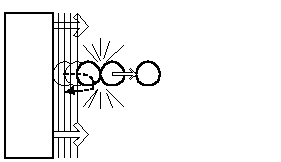Figure 2. Molecule motion during compression. The vibrating structure moves the molecule, which impacts adjoining molecules in a "chain reaction" motion. When the structure moves in the opposite direction, the molecules move back in a similar fashion, one following the other.

This wave can be visually depicted as shown in Figure 3. As the millions of molecules surrounding the vibrating structure squeeze together and then spread apart, the sound wave spreads from the source in all directions, alternating as low pressure and then high pressure transitions through space. When the molecules are squeezed, the pressure is high. When they are decompressed, the pressure is low. Under normal conditions, the pressure waves move at approximately 944 feet per second (the speed of sound). If we were to place a very sensitive pressure sensor in this path and measure the pressure changes, we could determine the magnitude of the sound wave. That is exactly what a microphone does. The greater the magnitude of the pressure fluctuations, the louder the sound is to our ears.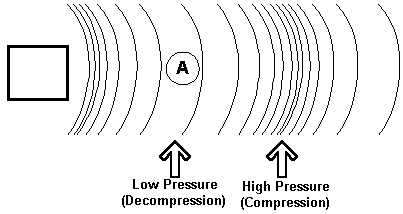Figure 3. The box is vibrating and causing molecules to compress and decompress in a chain reaction. These pressure changes move away from the box through space. If we were to stand a point "A", we would experience low pressure and high pressure waves move past us at the speed of sound.

Finally, the term acoustic impedance needs to be addressed. This concept is quite complex, but basically relates to the resistance that a sound pressure wave experiences when it travels across different materials and through different spaces. If the wave transitions from a space with one impedance into a different space with a different impedance, the wave loses energy and becomes weaker. This concept will be discussed in detail in the technical articles relating to horns and tonearms.

Frequency

One common element to both sound and vibration is the concept of frequency. In vibration, frequency is defined as the number of times a vibrating structure moves back and forth during one second. It is commonly measured in units of Hertz. A frequency of 1 Hertz means that the object moves back and forth only once during one second. A frequency of 1000 Hertz means that the object vibrates back and forth 1000 times per second. Subjectively, we hear lower frequencies as a lower pitch and higher frequency as higher pitch. In other words, bass notes are low frequencies and treble notes are high frequencies. For sound waves, frequency relates to the duration (in fractions of a second) that it takes for the passing sound waves to go from low pressure to high pressure and back to low pressure again. If a local vibrating structure is the source of a sound we hear, its vibrational frequency is the same as the frequency we hear through the sound wave. If we were standing at Point A in Figure 3 (above), we have already stated that the sound waves travel past us at 944 feet per second. If the structure is vibrating at low frequencies, the spacing between the compression and decompression parts of the sound wave would be much farther apart then it would be for high frequencies. To calculate the distance between the compression and decompression points in a soundwave (one-half wavelength) for any given frequency, the simple formula;

Distance (in feet) = 944/(2 x frequency)

Thus, for a 100 Hertz tone, the distance between the high pressure point and low pressure point is: 944/(2 x 100) = 944/200 = 4.72 feet

Thus, even though the sound wave (molecule-to-molecule impacts) moves past us at a fixed speed, the time it takes to go from low pressure to high pressure at a fixed point is dependent on the frequency of the source. This is because the distance (spacing) between the high and low pressure points varies as a function of frequency. Again, lower frequency sounds have more distance between the high and low pressure points in the sound wave. The number of pressure cycles that pass by the listener in one second is the frequency or pitch that we hear.

Human hearing has a limited range of response; an "ideal" ear can hear from approximately 20 Hertz up to 20,000 Hertz, but in reality, most people over the age of 20 have a hearing response in the range of 20-14,000 Hertz, rapidly decreasing with age. As a reference, the fundamental tone of A above middle C on a piano is 440 Hertz. When struck, the piano string vibrates at that frequency, and excites the nearby air molecules, causing a pressure wave of 440 Hertz to be passed to the listener. A doubling of the frequency is an octave. Thus, the next A on the piano (above the A440) has a fundamental frequency of 880 Hertz, an octave above.

The vast majority of sounds that we encounter in everyday life are in the range of 80 to 5000 Hertz. This includes the fundamental tones of most musical instruments. Sounds below 80 Hertz may be heard in the lowest notes of pipe organs or in the rumble of distant thunder. Sounds above 5000 Hertz are heard as the "sizzle" in cymbals or in some of the high overtones of a violin. If you play an orchestral recording through a high quality stereo system with an equalizer, you can experiment with the frequency content of the recording. Cutting out frequencies above 12,000 Hertz or so tends to reduce the subtle presence of the orchestra more than it affects the sound quality of the instruments themselves. Even when you cut out frequencies above 5,000 Hertz, most of the audio content of the orchestra is still present, although with much less sparkle or "life".

Amplitude

Another term that is common to both vibration and sound is the concept of amplitude. In vibration, amplitude refers to the maximum motion that a structure moves as it vibrates. Any motion can be defined in terms of displacement (inches), velocity (inches per second), or acceleration (g's). Refer to Figure 4. In this example, this tuning fork is vibrating. If we could use a imaginary magnifying glass, we could see the tiny motion of one of the fork's tines as it vibrates back and forth. In this case, the fork tine is moving left from center a small distance "Y" and right from center the same distance "Y" as it vibrates. If we were to track the motion of one of the tines as we moved it through space, we would end up with a "sine wave" pattern, as shown in the example. The sine wave has a distinctive shape. It's maximum amplitude (or peak value) is "Y", the same amount as the tine moves as it vibrates.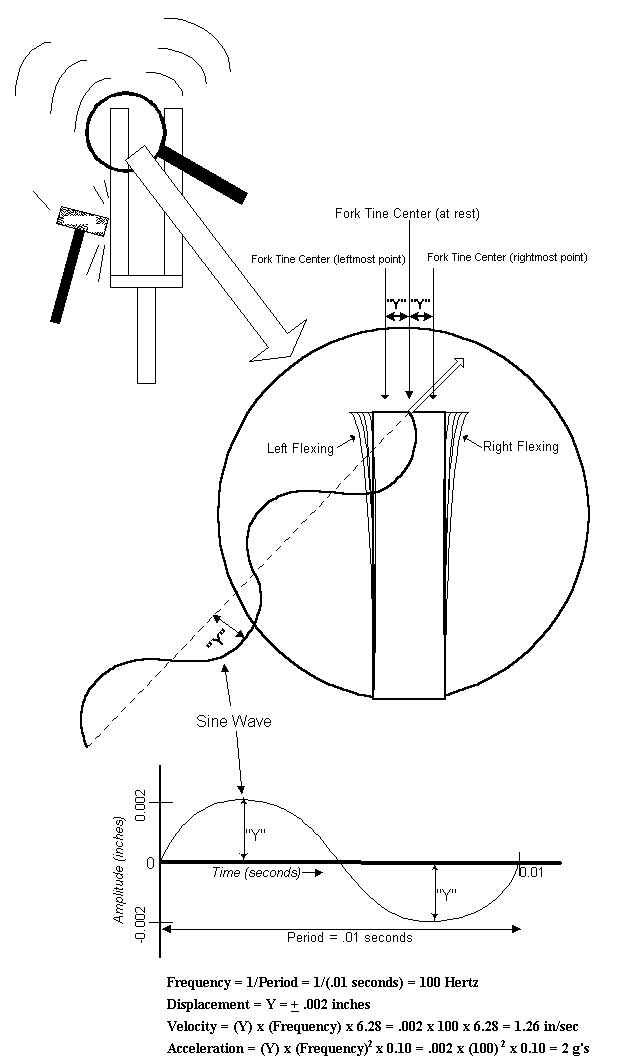Figure 4. The tuning fork vibrates back and forth. If we were to view this motion through a magnifying glass as we moved the fork through space, a sine wave would be produced. This wave can be evaluated to determine its displacement, speed or acceleration.

If we were to look "straight on" to this sine wave shape, we would see a curve as shown on the bottom of Figure 4. In this example, we used specialized measurement instruments to measure the amplitude (value of "Y") as being 0.002 inches (2/1000 inch). We also were able to measure the length of time that it takes for the sine wave to complete one cycle (move from rest in one direction, then the other direction, and back to the rest position again), and found it to be 0.01 seconds (1/100 second). This time is known as the period.

Once these simple parameters are measured, we can understand a great deal about the vibration of the tuning fork. For example, if we take the reciprocal of the period, we can determine the fork tine's frequency. In this example, the tine is vibrating at 100 Hertz. We already measured the amplitude of the displacement as being .002 inches. If we are interested, we can simply apply the equations shown at the bottom of the figure to calculate the tine's peak velocity (or speed) in inches per second as it vibrates. In this case, the tine vibrates at a peak speed of 1.26 inches per second. Alternatively, we could calculate its peak acceleration. The tine's peak acceleration is 2g's, twice the acceleration of gravity. Note from these equations, as the frequency rises, so does the velocity and acceleration; if the tine is vibrating back and forth more times in a second, its velocity has to increase accordingly. The important thing to bear in mind here is that any of these parameters (displacement, velocity or acceleration) can be used to describe vibration. Once we know the frequency of vibration and any one of these parameters, we can easily calculate the others using simple equations.

For sound waves, amplitude relates to the levels of maximum pressure fluctuations in the sound wave. The base unit for sound pressure is the Pascal. One Pascal is equal to approximately 0.000145 pounds per square inch (PSI). For example, a typical conversational voice generates approximately 0.063 Pascals of sound pressure. A whisper would generate 0.0011 Pascals, while a 747 jet during take-off could produce as much as 6,500 Pascals (0.95 PSI) of sound pressure. Using these pressure units to measure sound are somewhat unwieldy, since the human ear does not respond to sound pressure in a linear fashion. In other words, a sound pressure of 2 Pascals would not sound as twice as loud as a sound pressure of 1 Pascal. Thus, most practical measurements of sound levels use decibels as the units of measure. The decibel is a calculation based on taking the logarithm of the Pascal measurements, defined as:

dB = 20 log10 (Sound Pressure measured in Pascals / .00002)

The dB is used because it better corresponds to subjective human hearing response. Using these units, the typical conversation measures in at 70 decibels, the whisper is 35 decibels, and the 747 jet measures in at 170 decibels. The important thing to remember with decibels is that the subjective sound level doubles for each 10 decibel increase. In other words, 70 decibels sounds twice as loud as 60 decibels, 100 decibels sounds twice as loud as 90 decibels, etc. The use of A-weighted decibel measurements is more accurate for determining subjective sound levels because it takes the ear's frequency response characteristics into account.

Complex Waveforms

Most sound and music that we hear does not consist of simple tones. When multiple frequencies are present at one time, the result is a complex waveform. The sine wave shown in Figure 3 is a simple 100 Hertz tone. If you could hear it, it would sound similar to the effect one hears when blowing across the mouth of a large jug. However, if one were to look at the waveform of a harp string plotted against time, it would look similar to the bottom plot in Figure 5. This is due to the presence of harmonics in the harp's vibrations. Musical instruments, human voices and most sounds in nature have high harmonic content. Harmonics are even multiples of a fundamental frequency, and give musical instruments their sound quality; it makes a flute sound like a flute and a trumpet sound like a trumpet. For example, if a piano plays an "A" at 440 Hertz, it will have some amount of second and fourth (as well as many other) harmonics. That means that the piano will produce not only the 440 Hertz "A" tone, but also 880 Hertz (the second harmonic) and 1760 Hertz (the fourth harmonic) of that note. A trumpet playing the same "A" would have different harmonic content, and thus, while we hear an "A" coming from both instruments, but they sound different to the ear. When many instruments are playing at once, the waveforms can become infinitely complex, but they can always be broken down into many individual sine wave components for analysis.

Harmonics are always caused by some amount of flexing (distortion) in the vibrating structure. That would include the flexibility in a piano string, a trumpeter's lips, or in the human voicebox. But when components in sound reproducing equipment flex, they tend to
add additional (unwanted) harmonics to the reproduced sound, and we term this phenomena harmonic distortion.

A simple example of a complex waveform is seen in Figure 5. The upper plot shows a simple sine wave with a period of 0.002 seconds, or 500 Hertz. The second plot is a sine wave that completes 3 complete cycles in the same 0.002 seconds, so its period is .002/3 or 0.00067 seconds. This equates to a frequency of 1500 Hertz (3 times higher than the first plot). Thus, the second plot is the third harmonic of the first plot. If these two curves were combined, the complex waveform on the bottom would result.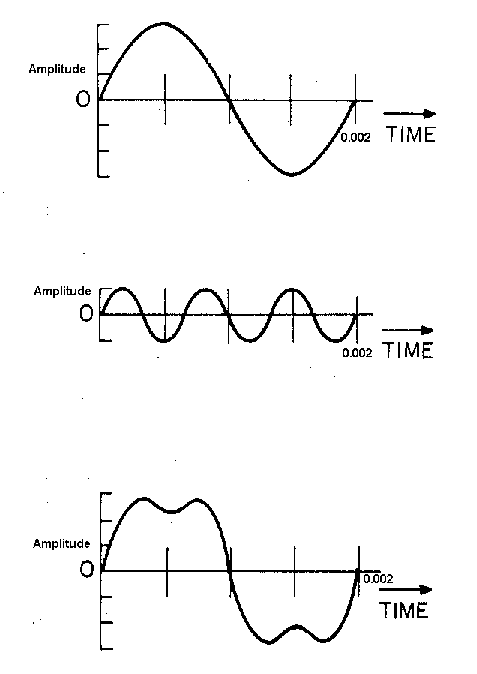Figure 5. The complex waveform on the bottom is the summation of the two simple waveforms above it.

Other complex waveforms are generated when a transient event occurs. A good example of a transient event would be the "click" heard on a phonograph record due to a scratch or other defect. The resulting waveform would be as seen in Figure 6. There is a sudden discontinuity in the waveform due to the sudden impact of the needle through the scratch. Analysis of this waveform might include the amplitude and duration of the transient event.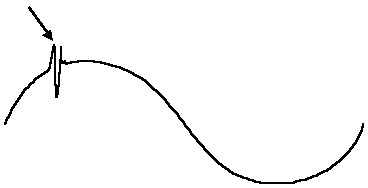Figure 6. The transient event (denoted by arrow) disrupts the "smooth" flow of the waveform.

Measurement Instrumentation

Many specialized instruments are available to study the effects of sound and vibration, and to measure and compare the performance of sound reproduction equipment. The microphone (mentioned above) is used to measure the sound pressure fluctuations at any point in the listening room. Small accelerometers can be attached to surfaces to measure the vibration of a structure, and can produce output signals proportional to the structure's acceleration, velocity or displacement. Laser beams can be pointed at vibrating objects and measure the amount of motion and frequency content of the vibrations.

But the "key" to evaluating all this data is the Signal Analyzer, a computerized instrument that allows the engineer to make comparative measurements, and evaluate these signals in an infinite number of combinations. Signals can be viewed in terms of amplitude or frequency. Displacements and pressure levels can be easily determined. When processed, this data can show the weak links and performance characteristics of sound reproduction equipment and components, and direct the analyst to the reasons why certain components produce certain sound qualities.

One of the most important tools that the acoustic analyst uses is the FFT, or Fast Fourier Transform technique. Basically, this process converts a sine wave into its frequency and amplitude components, represented as a single "bar" for each simple sine wave. If we were to look at the FFT measurements of the 3 sine waves in Figure 5, the results would appear as shown in Figure 7. The FFT analysis allows us to breakdown the simple (or highly complex) waveforms to determine the frequency and amplitude content in each waveform. This is a highly valuable aid in measuring and comparing the sound and vibrations from any source.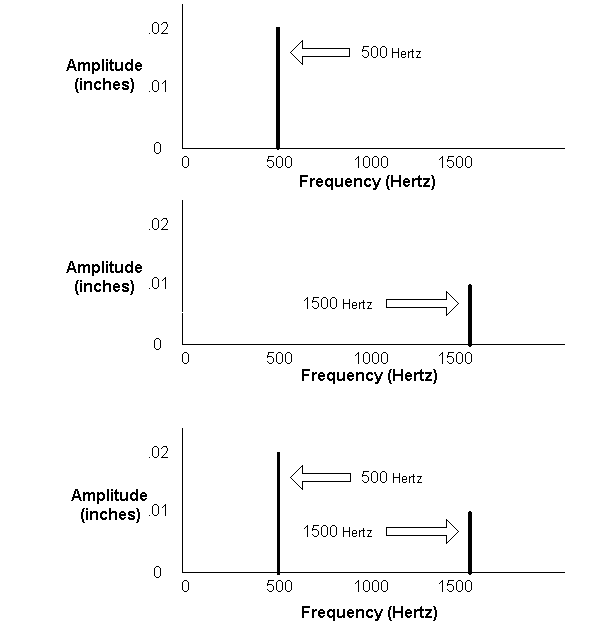Figure 7. FFT Representations of the sine wave plots of Figure 5 (above). The upper plot shows that the topmost sine wave has a frequency of 500 Hertz, and an amplitude of .02 inches. The middle plot shows that the center sine wave has a frequency of 1500 Hertz (3 times the frequency of the top sine wave) and an amplitude of .01 inches (one half the amplitude of the top sine wave). The bottom FFT shows the breakdown of the complex bottom sine wave as being the sum of the two individual sine waves. In this manner, the frequency and amplitude of any waveform can be easily evaluated.